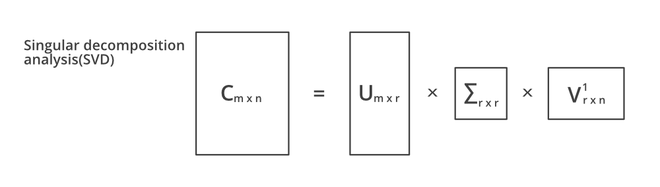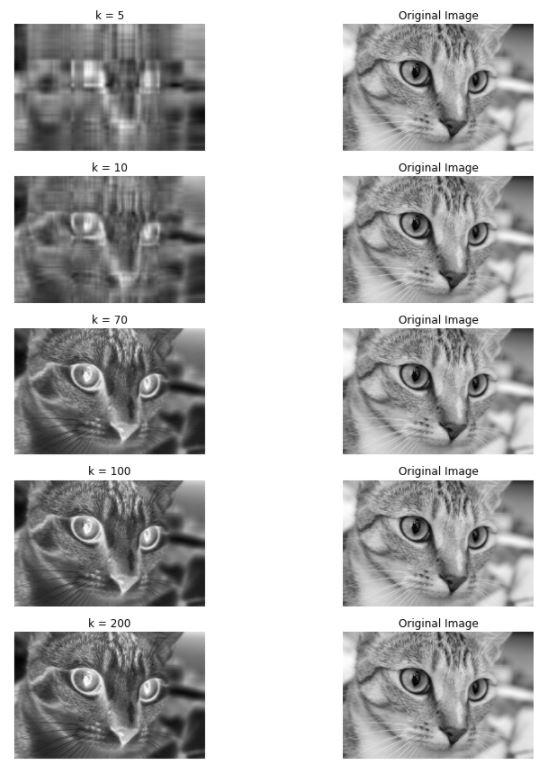# Singular Value Decomposition (SVD)

• Last Updated : 19 Nov, 2021

The Singular Value Decomposition (SVD) of a matrix is a factorization of that matrix into three matrices. It has some interesting algebraic properties and conveys important geometrical and theoretical insights about linear transformations. It also has some important applications in data science. In this article, I will try to explain the mathematical intuition behind SVD and its geometrical meaning.

#### Mathematics behind SVD

The SVD of  mxn matrix A is given by the formula :

Attention reader! Don’t stop learning now. Get hold of all the important Machine Learning Concepts with the Machine Learning Foundation Course at a student-friendly price and become industry ready.where:

• U:  mxn matrix of the orthonormal eigenvectors of.
• VT: transpose of a nxn matrix containing the orthonormal eigenvectors of A^{T}A.
• W:  a nxn diagonal matrix of the singular values which are the square roots of the eigenvalues of.#### Examples

• Find the SVD for the matrix A =• To calculate the SVD, First, we need to compute the singular values by finding eigenvalues of AA^{T}.• The characteristic equation for the above matrix is:so our singular values are:• Now we find the right singular vectors i.e orthonormal set of eigenvectors of ATA. The eigenvalues of ATA are 25, 9, and 0, and since ATA is symmetric we know that the eigenvectors will be orthogonal.

Forwhich can be row-reduces to :A unit vector in the the direction of it is:Similarly, for \lambda = 9, the eigenvector is:For the 3rd eigenvector, we could use the property that it is perpendicular to v1 and v2 such that:Solving the above equation to generate the third eigenvectorNow, we calculate U using the formula u_i = \frac{1}{\sigma} A v_i and this gives U =. Hence, our final SVD equation becomes:### Applications

• Calculation of Pseudo-inverse:  Pseudo inverse or Moore-Penrose inverse is the generalization of the matrix inverse that may not be invertible (such as low-rank matrices). If the matrix is invertible then its inverse will be equal to Pseudo inverse but pseudo inverse exists for the matrix that is not invertible. It is denoted by A+.

Suppose, we need to calculate the pseudo-inverse of a matrix M:

Then, the SVD of M can be given as:Multiply both sides by M^{-1}.Multiply both side by V:Multiply by W^{-1}. Since the W is the singular matrix, the inverse of WisMultiply byThe above equation gives the pseudo-inverse.

• Solving a set of Homogeneous Linear Equation (Mx =b): if b=0,  calculate SVD and take any column of VT associated with a singular value (in W) equal to 0.

IfMultiply byFrom the Pseudo-inverse, we know thatHence,• Rank, Range, and Null space:
• The rank of matrix M can be calculated from SVD by the number of nonzero singular values.
• The range of matrix M is The left singular vectors of U corresponding to the non-zero singular values.
• The null space of matrix M is The right singular vectors of V corresponding to the zeroed singular values.• Curve Fitting Problem: Singular value decomposition can be used to minimize the least square error. It uses the pseudo inverse to approximate it.
• Besides the above application, singular value decomposition and pseudo-inverse can also be used in Digital signal processing and image processing

### Implementation

• In this code, we will try to calculate the Singular value decomposition using Numpy and Scipy.  We will be calculating SVD, and also performing pseudo-inverse. In the end, we can apply SVD for compressing the image

## Python3

 # Imports import numpy as npfrom scipy.linalg import svd """Singular Value Decomposition"""# define a matrixX = np.array([[3, 3, 2], [2,3,-2]])print(X)# perform SVDU, singular, V_transpose = svd(X)# print different componentsprint("U: ",U)print("Singular array",s)print("V^{T}",V_transpose) """Calculate Pseudo inverse"""# Onverse of singular matrix is just the reciprocal of each elementsingular_inv = 1.0 / singular# create m x n matrix of zeroes and put singular values in its_inv = np.zeros(A.shape)s_inv= singular_invs_inv =singular_inv# calculate pseudoinverseM = np.dot(np.dot(V_transpose.T,s_inv.T),U.T)print(M) """SVD on image compression""" import numpy as npimport matplotlib.pyplot as pltfrom skimage import datafrom skimage.color import rgb2gray cat = data.chelsea()plt.imshow(cat)# convert to grayscalegray_cat = rgb2gray(cat) # calculate the SVD and plot the imageU,S,V_T = svd(gray_cat, full_matrices=False)S = np.diag(S)fig, ax = plt.subplots(5, 2, figsize=(8, 20)) curr_fig=0for r in [5, 10, 70, 100, 200]:  cat_approx =U[:, :r] @  S[0:r, :r] @ V_T[:r, :]  ax[curr_fig].imshow(256-cat_approx)  ax[curr_fig].set_title("k = "+str(r))  ax[curr_fig,0].axis('off')  ax[curr_fig].set_title("Original Image")  ax[curr_fig].imshow(gray_cat)  ax[curr_fig,1].axis('off')  curr_fig +=1plt.show()

Output:

[[ 3  3  2]
[ 2  3 -2]]
---------------------------
U:  [[-0.7815437 -0.6238505]
[-0.6238505  0.7815437]]
---------------------------
Singular array [5.54801894 2.86696457]
---------------------------
V^{T} [[-0.64749817 -0.7599438  -0.05684667]
[-0.10759258  0.16501062 -0.9804057 ]
[-0.75443354  0.62869461  0.18860838]]
--------------------------
# Inverse
array([[ 0.11462451,  0.04347826],
[ 0.07114625,  0.13043478],
[ 0.22134387, -0.26086957]])
---------------------------Original vs SVD k-image

## References:

My Personal Notes arrow_drop_up